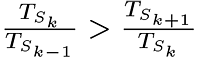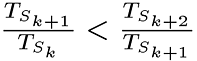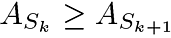Next:Application of the analysesUp:Constructing a Domain Model Previous:The Paradigmatic Approach

## Harris's Tree Structure Analysis

The purpose of the Harris's tree structure analysis is to provide another way to find the strongest joints between sequential terms in the corpus. This analysis is based on a method for discovering morpheme boundaries from sentence-forming phoneme sequences . A tree structure is formed that branches when differences occur. Kimmo Koskenniemi  describes a slightly modified tree structure, where morphemes (words), are assigned to branches and the amounts of texts that are identical so far, to nodes.

He describes also formation of a syntax tree for a morpheme sequence based on

• the numbers of sequences that are equal to each point, and
• the numbers of possible continuations after each morpheme.
The morpheme sequence might actually be an English sentence in a corpus.

Supposing that

(a)
$S$1,S2,...Sn are the morphemes (words) in a sequence (sentence),
(b)
$TS$k is the number of sequences equal up to the morpheme$S$k, and
(c)
$AS$k is the number of different successors of morpheme $S$k-1
the morphemes $S$k and $S$k+1 are supposed to be joint in the syntax tree for the sequence if
(a),
(b), and
(c).

The most interesting parts of the syntax tree are the strongest pairs of words at the lowest level.Next:Application of the analysesUp:Constructing a Domain Model Previous:The Paradigmatic Approach
Päivikki Parpola

Sat Oct 14 22:52:14 EEST 2000